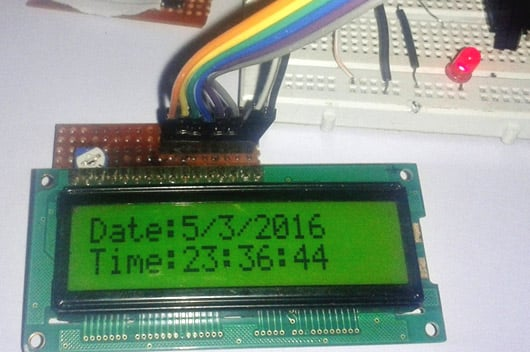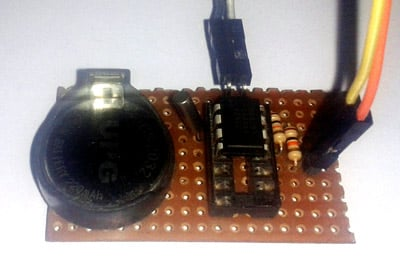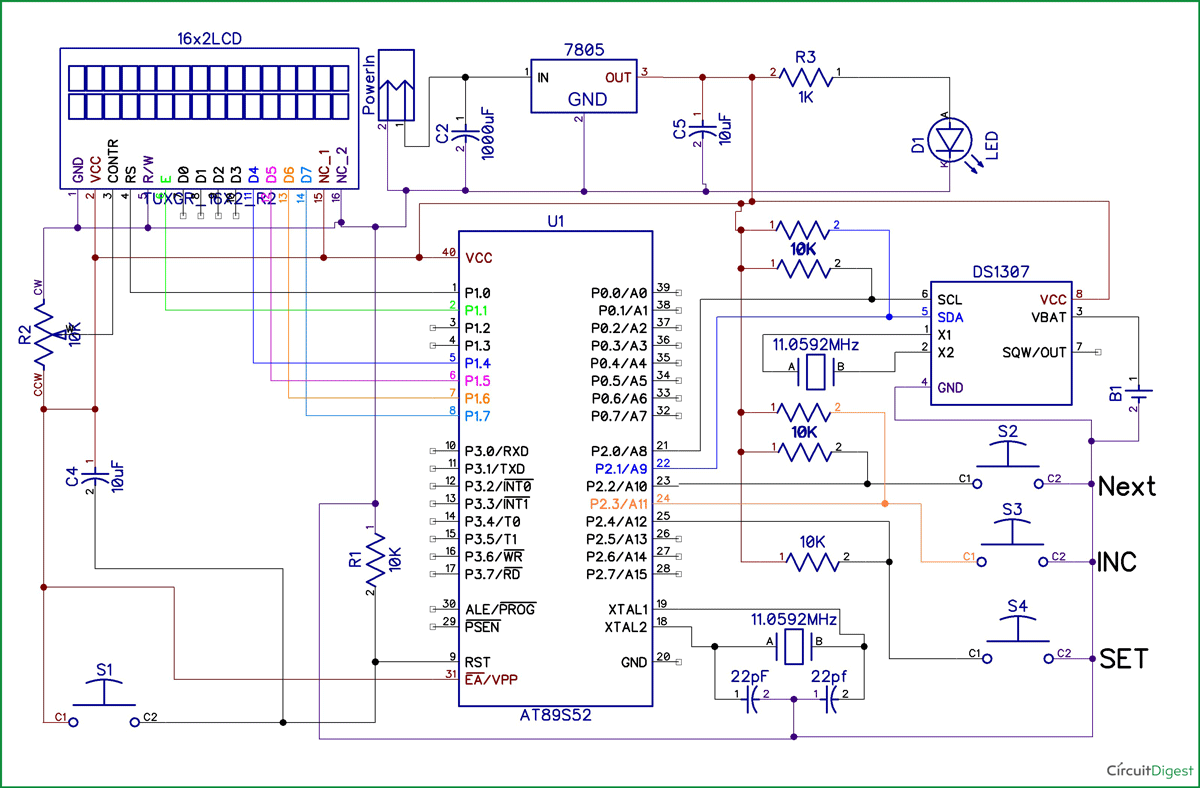# Digital Clock using 8051 Microcontroller

Published  March 14, 2016   12
S
AuthorIn this project, we are going to demonstrate making a RTC Clock using 8051 microcontroller.  If you would like to do this project with Arduino, check this digital clock using Arduino. The major component of this project is DS1307 which is a real time digital clock IC. Lets know about this IC in detail.

RTC DS1307:

The DS1307 serial real-time clock (RTC) is a low-power, full binary-coded decimal (BCD) clock/calendar plus 56 bytes of NV SRAM. This chip works on I²C protocol. The clock/calendar provides seconds, minutes, hours, day, date, month, and year information. The end of the month date is automatically adjusted for months with fewer than 31 days, including corrections for leap year. The clock operates in either the 24-hour or 12-hour format with AM/PM indicator. The DS1307 has a built-in power-sense circuit that detects power failures and automatically switches to the backup supply. Timekeeping operation continues while the part operates from the backup supply. DS1307 chip can continuously run till 10 year.8051 based Real time clock is a digital clock to display real time using a RTC DS1307, which works on I2C protocol. Real time clock means it runs even after power failure. When power is reconnected, it displays the real time irrespective to the time and duration it was in off state. In this project we have used a 16x2 LCD module to display the time in - (hour, minute, seconds, date, month and year) format. Real time clocks are commonly used in our computers, houses, offices and electronics device for keeping them updated with real time.

I2C protocol is a method to connect two or more devices using two wires to a single system, and so this protocol is also called as two wire protocol. It can be used to communicate 127 devices to a single device or processor. Most of I2C devices run on 100Khz frequency.

Steps for data write master to slave (slave receiving mode)

1. Sends START condition to slave.
2. Sends slave address to slave.
3. Send write bit (0) to slave.
4. Received ACK bit from slave
5. Sends words address to slave.
6. Received ACK bit from slave
7. Sends data to slave.
8. Received ACK bit from slave.
9. And last sends STOP condition to slave.

Steps for data reading from slave to master (slave transmitting mode)

1. Sends START condition to slave.
2. Sends slave address to slave.
3. Send read bit (1) to slave.
4. Received ACK bit from slave
6. Received ACK bit from slave.
7. Sends  STOP condition to slave.

### Circuit Diagram and Description

In circuit we have used 3 most components DS1307, AT89S52 and LCD. Here AT89S52 is used for reading time from DS1307 and display it on 16x2 LCD screen. DS1307 sends time/date using 2 lines to the microcontroller.Circuit connections are simple to understand and shown in the above diagram. DS1307 chip pin SDA and SCL are connected to P2.1 and P2.0 pins of 89S52 microcontroller with pull up resistor that holds default value HIGH at data and clock lines. And a 32.768KHz crystal oscillator is connected with DS1307chip for generating exact 1 second delay. And a 3 volt battery is also connected to pin 3rd (BAT) of DS1307 which keeps time running after electricity failure. 16x2 LCD is connected with 8051 in 4-bit mode. Control pin RS, RW and En are directly connected to 89S52 pin P1.0, GND and P1.1. And data pin D0-D7 is connected to P1.4-P1.7 of 89S52.

Three buttons namely SET, INC/CHANGE and Next are used for setting clock time to pin P2.4, P2.3 and P2.2 of 89S52 (active low). When we press SET, time set mode activates and now we need to set time by using INC/CHANGE button and Next button is used for moving to digit. After setting time clock runs continuously.

### Program Description

In code we have included 8051 family library and a standard input output library. And defined pins that we have used, and taken some variable for calculations.

```#include<reg51.h>
#include<stdio.h>
#define lcdport P1

sbit rs=P1^0;
sbit en=P1^1;

sbit SDA=P2^1;
sbit SCL=P2^0;

sbit next=P2^2;                     //increment digit
sbit inc=P2^3;                     //increment value
sbit set=P2^4;                     //set time

char ack;
unsigned char day1=1;
unsigned char k,x;
unsigned int date=1, mon=1, hour=0, min=0, sec=0;
int year=0;

void delay(int itime)
{
int i,j;
for(i=0;i<itime;i++)
for(j=0;j<1275;j++);
}```

And the given function is used for driving LCD.

```void daten()
{
rs=1;
en=1;
delay(1);
en=0;
}

void lcddata(unsigned char ch)
{
lcdport=ch & 0xf0;
daten();
lcdport=(ch<<4) & 0xf0;
daten();
}

void cmden(void)
{
rs=0;
en=1;
delay(1);
en=0;
}

void lcdcmd(unsigned char ch)```

This function is used for initialise RTC and and read time and date from form RTC IC.

```I2CStart();
I2CSend(0xD0);
I2CSend(0x00);
I2CStart();
I2CSend(0xD1);
I2CStop();
show_time();                                           //display time/date/day
delay(1);```

These functions is used for converting decimal to BCD and BCD to Decimal.

```int BCDToDecimal(char bcdByte)
{
char a,b,dec;
a=(((bcdByte & 0xF0) >> 4) * 10);
b=(bcdByte & 0x0F);
dec=a+b;
return dec;
}

char DecimalToBCD (int decimalByte)
{
char a,b,bcd;
a=((decimalByte / 10) << 4);
b= (decimalByte % 10);
bcd=a|b;
return bcd;
}```

The given below functions is used for I2C Communication.

```void I2CStart(){SDA=1;SCL=1,SDA=0,SCL=0;}             //"start" function for communicate with ds1307 RTC
void I2CStop(){SDA=0,SCL=1,SDA=1;}                     //"stop" function for communicate wit ds1307 RTC

unsigned char I2CSend(unsigned char Data)             //send data to ds1307
{
char i;
char ack_bit;
for(i=0;i<8;i++)
{
if(Data & 0x80) SDA=1;
else SDA=0;
SCL=1;
Data<<=1;
SCL=0;
}
SDA=1,SCL=1;
ack_bit=SDA;
SCL=0;
return ack_bit;
}

{
unsigned char i, Data=0;
SDA=1;
for(i=0;i<8;i++)
{
Data<<=1;
do{SCL=1;}
while(SCL==0);
if(SDA) Data|=1;
SCL=0;
}
if(ack)SDA=0;
else SDA=1;
SCL=1;
SCL=0;
SDA=1;
return Data;
}```

The set_time function is used to set the time in clock and show_time function below is used to display time on LCD.

``` void show_time()                            //function to display time/date/day on LCD
{
char var;
lcdcmd(0x80);
lcdprint("Date:");
sprintf(var,"%d",date);
lcdprint(var);
sprintf(var,"/%d",mon);
lcdprint(var);
sprintf(var,"/%d",year+2000);
lcdprint(var);
lcdprint("   ");
lcdcmd(0xc0);
lcdprint("Time:");
sprintf(var,"%d",hour);
lcdprint(var);
sprintf(var,":%d",min);
lcdprint(var);
sprintf(var,":%d",sec);
lcdprint(var);
lcdprint(" ");
// day(day1);
lcdprint("   ");
}```
Code

#include<reg51.h>
#include<stdio.h>
#define lcdport P1

sbit rs=P1^0;
sbit en=P1^1;

sbit SDA=P2^1;
sbit SCL=P2^0;

sbit next=P2^2;                     //increment digit
sbit inc=P2^3;                     //increment value
sbit set=P2^4;                     //set time

char ack;
unsigned char day1=1;
unsigned char k,x;
unsigned int date=1, mon=1, hour=0, min=0, sec=0;
int year=0;

void delay(int itime)
{
int i,j;
for(i=0;i<itime;i++)
for(j=0;j<1275;j++);
}

void daten()
{
rs=1;
en=1;
delay(1);
en=0;
}

void lcddata(unsigned char ch)
{
lcdport=ch & 0xf0;
daten();
lcdport=(ch<<4) & 0xf0;
daten();
}

void cmden(void)
{
rs=0;
en=1;
delay(1);
en=0;
}

void lcdcmd(unsigned char ch)
{
lcdport=ch & 0xf0;
cmden();
lcdport=(ch<<4) & 0xf0;
cmden();
}

void lcdprint(char *str)
{
while(*str)
{
lcddata(*str);
str++;
}
}

void lcd_init(void)
{
lcdcmd(0x02);
lcdcmd(0x28);
lcdcmd(0x0c);
lcdcmd(0x01);
}

void I2CStart(){SDA=1;SCL=1,SDA=0,SCL=0;}             //"start" function for communicate with ds1307 RTC
void I2CStop(){SDA=0,SCL=1,SDA=1;}                     //"stop" function for communicate wit ds1307 RTC

unsigned char I2CSend(unsigned char Data)             //send data to ds1307
{
char i;
char ack_bit;
for(i=0;i<8;i++)
{
if(Data & 0x80) SDA=1;
else SDA=0;
SCL=1;
Data<<=1;
SCL=0;
}
SDA=1,SCL=1;
ack_bit=SDA;
SCL=0;
return ack_bit;
}

{
unsigned char i, Data=0;
SDA=1;
for(i=0;i<8;i++)
{
Data<<=1;
do{SCL=1;}
while(SCL==0);
if(SDA) Data|=1;
SCL=0;
}
if(ack)SDA=0;
else SDA=1;
SCL=1;
SCL=0;
SDA=1;
return Data;
}

/*void day(char d)                                // Function for display day on LCD
{
switch(d)
{
case 0:
lcdprint("DAY");
break;

case 1:
lcdprint("SUN");
break;

case 2:
lcdprint("MON");
break;

case 3:
lcdprint("TUE");
break;

case 4:
lcdprint("WED");
break;

case 5:
lcdprint("THU");
break;

case 6:
lcdprint("FRI");
break;

case 7:
lcdprint("SAT");
break;
}
}     */

int BCDToDecimal(char bcdByte)
{
char a,b,dec;
a=(((bcdByte & 0xF0) >> 4) * 10);
b=(bcdByte & 0x0F);
dec=a+b;
return dec;
}

char DecimalToBCD (int decimalByte)
{
char a,b,bcd;
a=((decimalByte / 10) << 4);
b= (decimalByte % 10);
bcd=a|b;
return bcd;
}

void show_time()                            //function to display time/date/day on LCD
{
char var;
lcdcmd(0x80);
lcdprint("Date:");
sprintf(var,"%d",date);
lcdprint(var);
sprintf(var,"/%d",mon);
lcdprint(var);
sprintf(var,"/%d",year+2000);
lcdprint(var);
lcdprint("   ");
lcdcmd(0xc0);
lcdprint("Time:");
sprintf(var,"%d",hour);
lcdprint(var);
sprintf(var,":%d",min);
lcdprint(var);
sprintf(var,":%d",sec);
lcdprint(var);
lcdprint(" ");
// day(day1);
lcdprint("   ");
}

void set_time()                                            //time set function
{
lcdcmd(0x0e);
while(k<7)
{
while(k==3)                                            //set date
{
x=year%4;
if(inc==0)
{date++;while(inc==0);
if(x==1 && mon==2 && date==28){date=1;}                //check for 28 day febuary
if(x==0 && mon==2 && date==29){date=1;}                //check for 29 day febuary
if((date==31) && (mon==4) || (mon==6) || (mon==9) || (mon==17)){date=1;}        // check for 30 day month
if(date==32){date=1;}                                                        // check for 31 day month
show_time();}
if(next==0)
{
k=5;

while(next==0);
}                                                //check for next digit
lcdcmd(0x85);
}

while(k==2)                                            //set month
{
if(inc==0)
{mon++;while(inc==0);
if(mon==13){mon=1;}                                  //check for end of year
show_time(); }
if(next==0){k=3;
while(next==0);

}
lcdcmd(0x88);
}

while(k==1)                                         //set year
{
if(inc==0)
{year++;while(inc==0);
if(year==30){year=0;}
show_time();     }
if(next==0){k=2;

while(next==0);}
lcdcmd(0x8d);
}

while(k==5)                                      //set hour
{
if(inc==0)
{hour++;while(inc==0);
if(hour==24){hour=0;}
show_time();}
if(next==0){k=6;
while(next==0);}
lcdcmd(0xc5);
}

while(k==6)                                       //set min
{
if(inc==0)
{min++;while(inc==0);
if(min==60){min=0;}
show_time();}
if(next==0){k=10;
while(next==0);}
lcdcmd(0xc8);
}
}

void main()
{
lcd_init();
lcdprint("Digital Clock");
lcdcmd(0xc0);
lcdprint(" Using 8051  ");
delay(400);
lcdcmd(1);
lcdprint("Circuit Digest");
lcdcmd(192);
delay(400);
while(1)
{
if(set==0)                                     // check time set button press
{
I2CStart();
I2CSend(0xD0);
I2CSend(0x00);
I2CSend(0x00);
I2CSend(0x00);
I2CSend(0x00);
I2CSend(0x01);
I2CSend(0x01);
I2CSend(0x01);
I2CSend(0x00);
I2CSend(0x80);
I2CStop();
k=1;
set_time();                     // call time set function
min=DecimalToBCD(min);
sec=DecimalToBCD(0);
hour=DecimalToBCD(hour);
year=DecimalToBCD(year);
mon=DecimalToBCD(mon);
date=DecimalToBCD(date);
I2CStart();
I2CSend(0xD0);
I2CSend(0x00);
I2CSend(0x00);
I2CSend(min);
I2CSend(hour);
I2CSend(day1);
I2CSend(date);
I2CSend(mon);
I2CSend(year);
I2CSend(0x80);
I2CStop();
lcdcmd(1);
lcdcmd(0x0c);
}

I2CStart();
I2CSend(0xD0);
I2CSend(0x00);
I2CStart();
I2CSend(0xD1);
I2CStop();
show_time();                                           //display time/date/day
delay(1);
}
}

Video

Tags

Submitted by Jaydas on Sun, 01/15/2017 - 20:21

### This code is working

This code is working
but display shows 2 seprate time and date and it exchange very fast

Submitted by Abubakar on Sat, 02/11/2017 - 23:25

### tnx for me sir

tnx for me sir

Submitted by Mohan on Tue, 03/14/2017 - 10:36

### Pls help me sir

sir i set date and time but at seconds when i press next button then again display shows default date and time 5/5/15 and 5/5/5..
it will not updated..
pls help me sir

### Im too facing the same

Im too facing the same problem

### Ans

Hi, 4nd

void I2CStart()
{
SDA=1;
SCL=1;
SDA=0;
}
void I2CStop()
{

SDA=0;
SCL=1;
SDA=1;
}

Submitted by Pawan Rewatkar on Tue, 06/13/2017 - 08:59

### Where is main code for this?

Where is main code for this? Actually m trying with this code but no character display on LCD. Plzz give some solution..

Submitted by Umamaheswari G… on Tue, 07/25/2017 - 00:42

### Hi,

Hi,

Where can I find required components for this project and where can I get those components, any website...

Thanks

Submitted by M.sridevi on Thu, 09/07/2017 - 21:23

### program for digital clock

program for digital clock using 7segBCD in 8051 microcontroller

Submitted by Lakhya on Sat, 10/21/2017 - 21:54

### Once the circuit is switched off..it goes back to its default

The problem i am facing is once the circuit is switched off and then when it is switched on again,it goes back to a default date n time.i have a 3v li battery attached too.what should i do?

Submitted by james on Tue, 12/12/2017 - 16:22